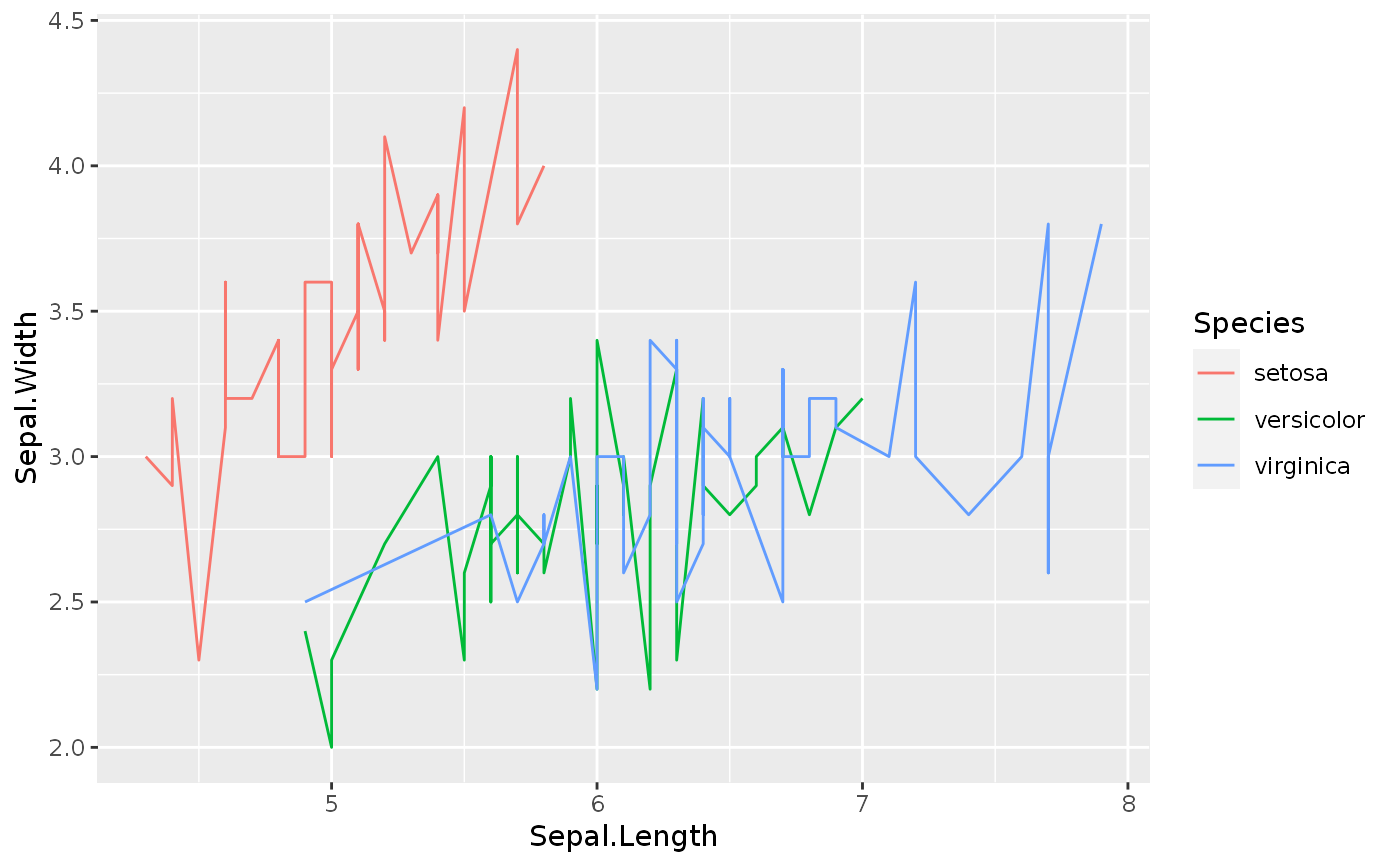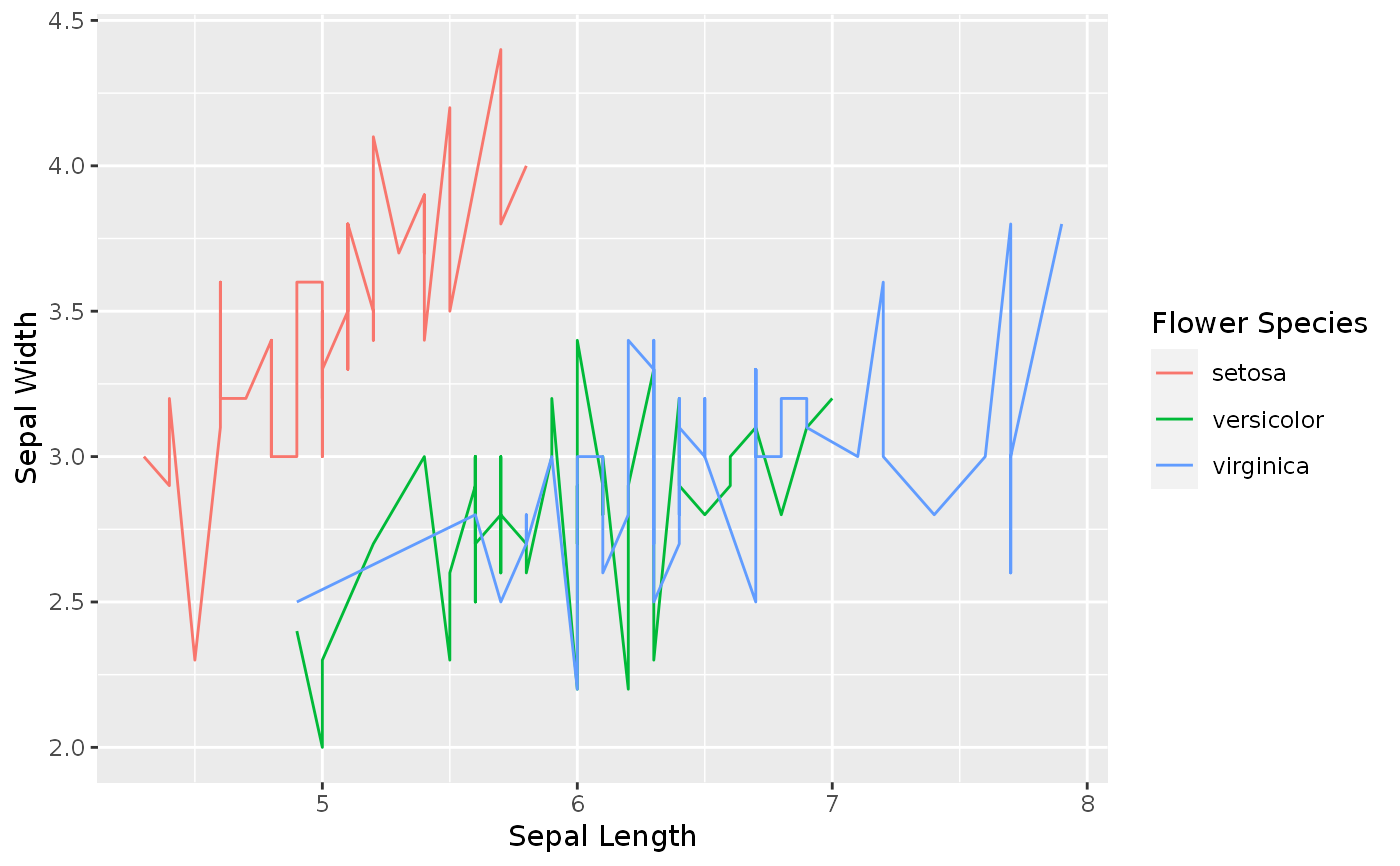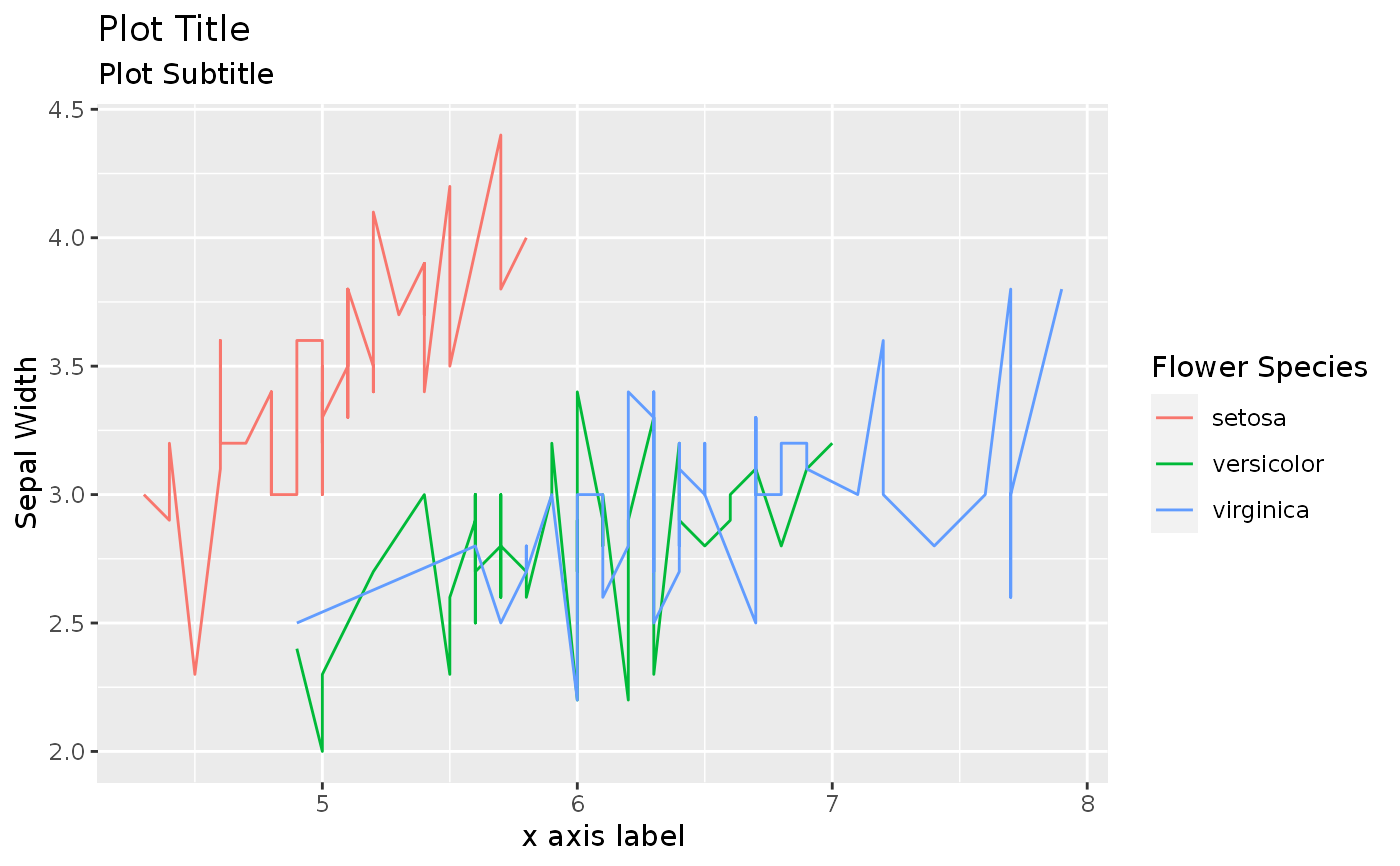Applies same logic as labs but uses as default the column label attribute if present as the variable label in the plot.

easy_labs(..., teach = FALSE)

## Arguments

... A list of new name-value pairs. The name should either be an aesthetic, or one of "title", "subtitle", or "caption" print longer equivalent ggplot2 expression?

## Examples


iris_labs <- iris

lbl <- c('Sepal Length', 'Sepal Width', 'Petal Length', 'Petal Width', 'Flower Species')

labelled::var_label(iris_labs) <- split(lbl,names(iris_labs))

p <- ggplot2::ggplot(iris_labs,ggplot2::aes(x=Sepal.Length,y=Sepal.Width))+
ggplot2::geom_line(ggplot2::aes(colour=Species))

pp + easy_labs()p + easy_labs(title = "Plot Title", subtitle = 'Plot Subtitle', x = 'x axis label')p + easy_labs(teach = TRUE)
#> easy_labs call can be substituted with:#> labs(x = 'Sepal Length', y = 'Sepal Width', colour = 'Flower Species')